# OECD Social spending data analysis 3 - Data Visualization with 5 Named Graphs (5NG) using RUnsplashAlicia Steelsが撮影した写真

This post is following of above post.
In the previous post, I made a dataframe for data analysis, named 'df2'.
Now, let's start data analysis with data visualization.
I will make 5 Named Graphs (5NG) using R.
My text book is

Chapter 2 Data Visualization | Statistical Inference via Data Science (moderndive.com)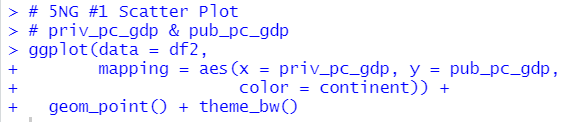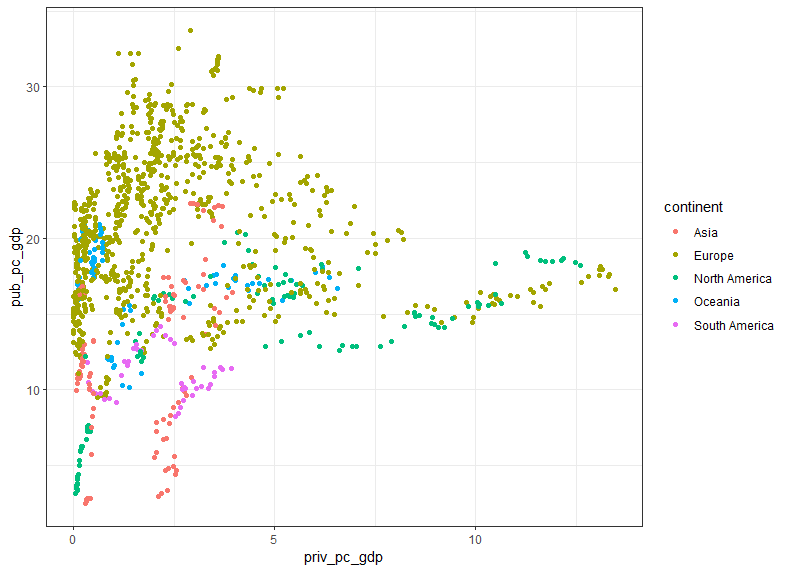geom_point() makes scatter plot. I see relatively positive relationship between priv_pc_gdp and pub_pc_gdp.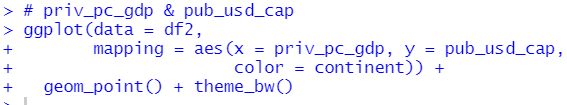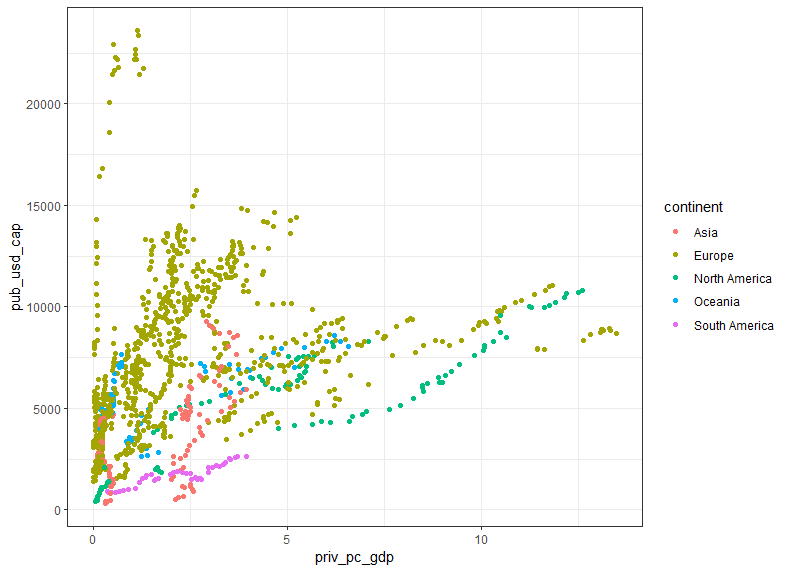I see relatively positive relationship between priv_pc_gdp and pub_usd_cap too.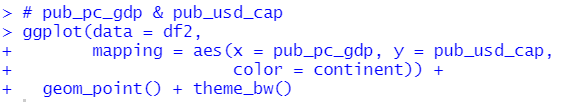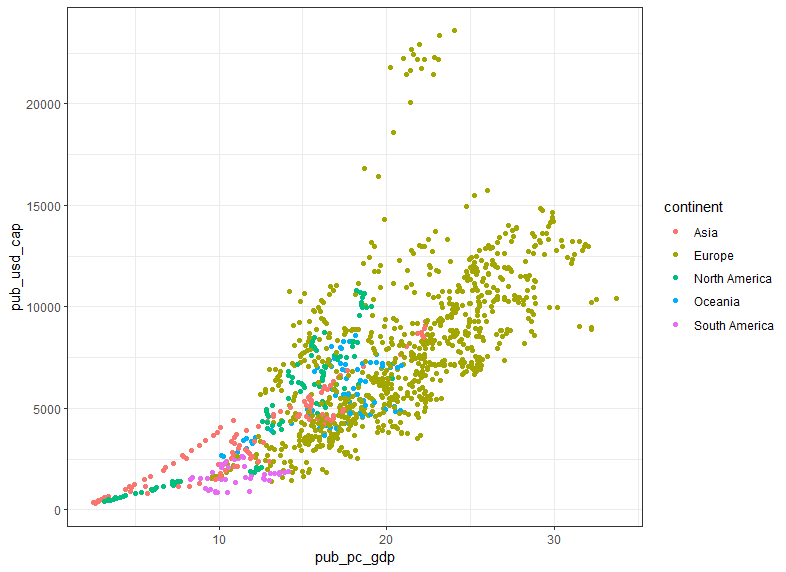I see there is strong correlation between pub_pc_gdp and pub_usd_cap.

Next, Line Graph.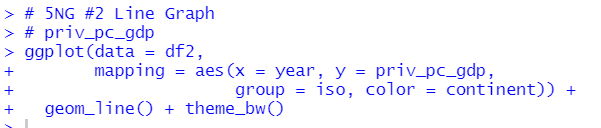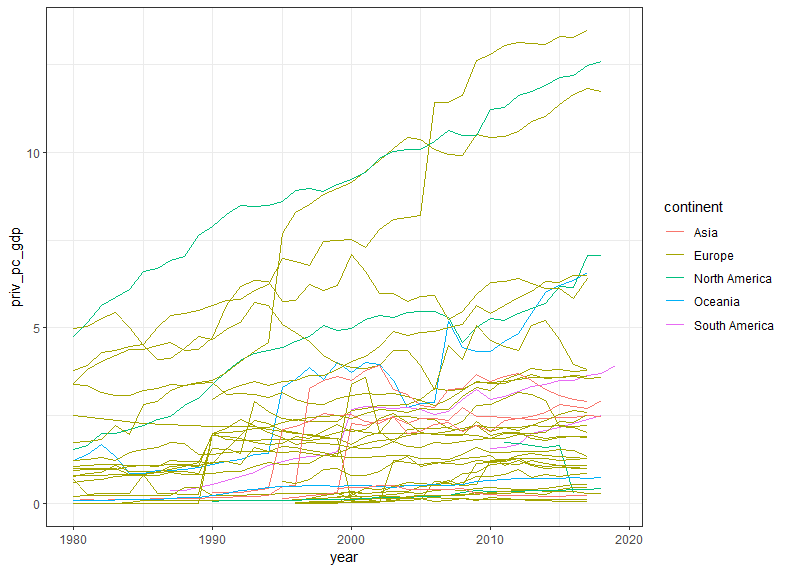geom_line() makes line graphs. prive_pc_gdp are increasing in general.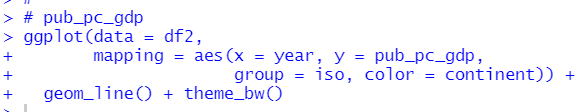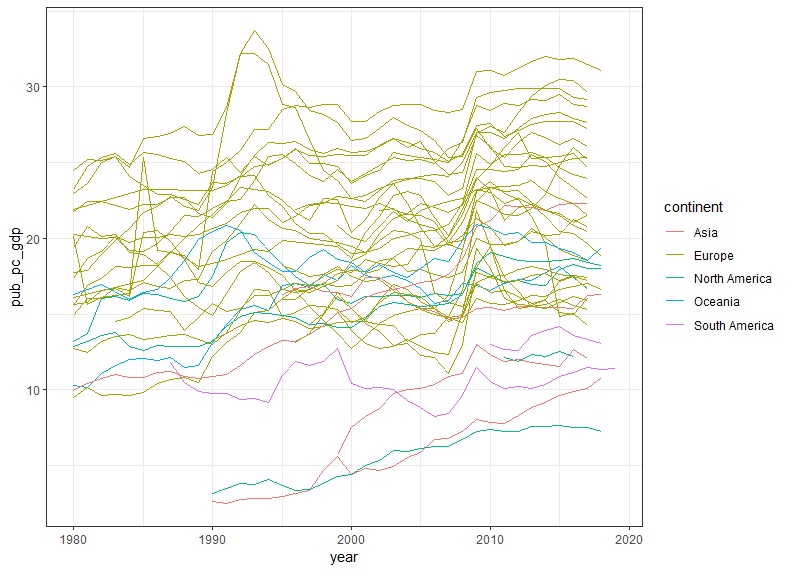pub_pc_gdp has more variation than priv_pc_gdp.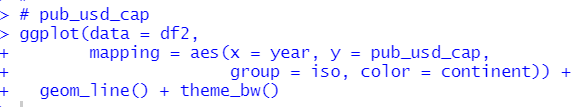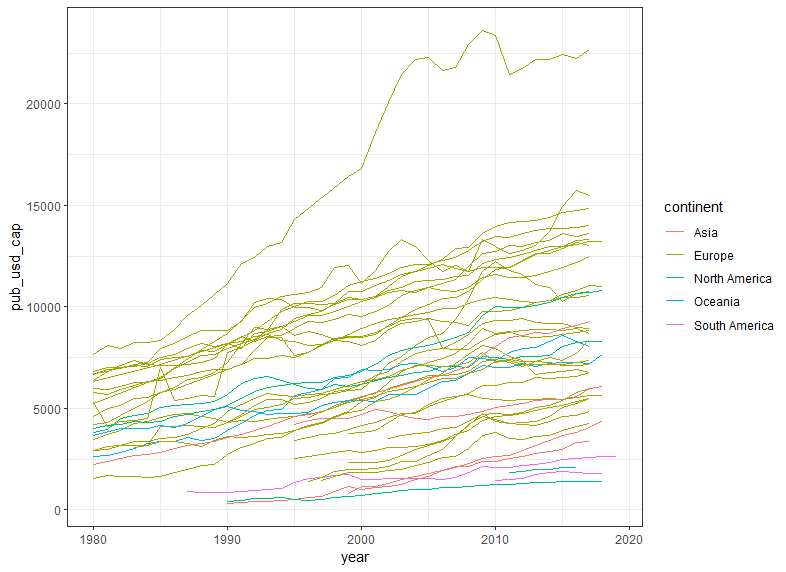pub_usd_cap are increasing for almost all countires.

#3 5NG is histogram.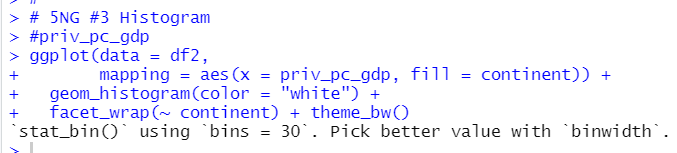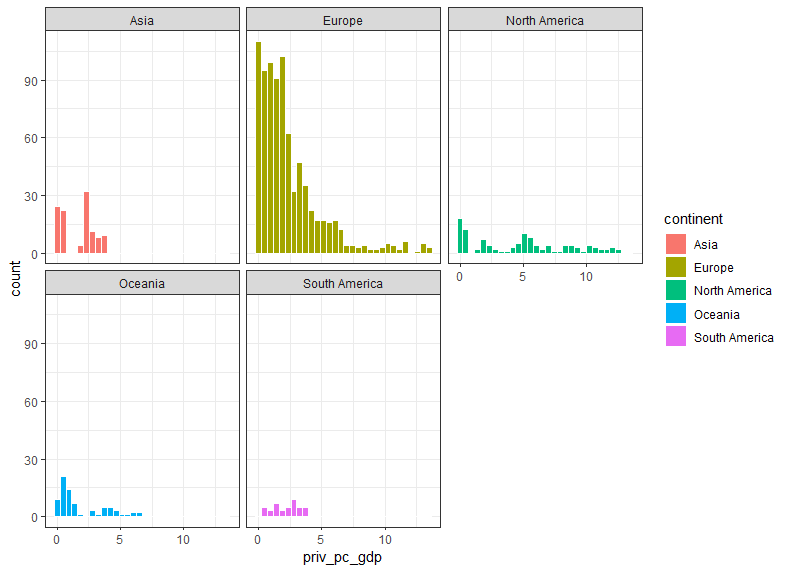geom_histogram() makes histograms. I use facet_wrap(~ continent) to make histograms for each continent.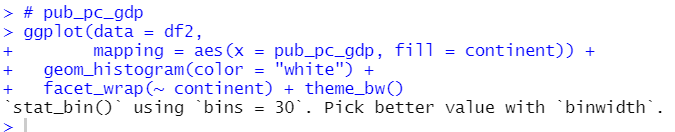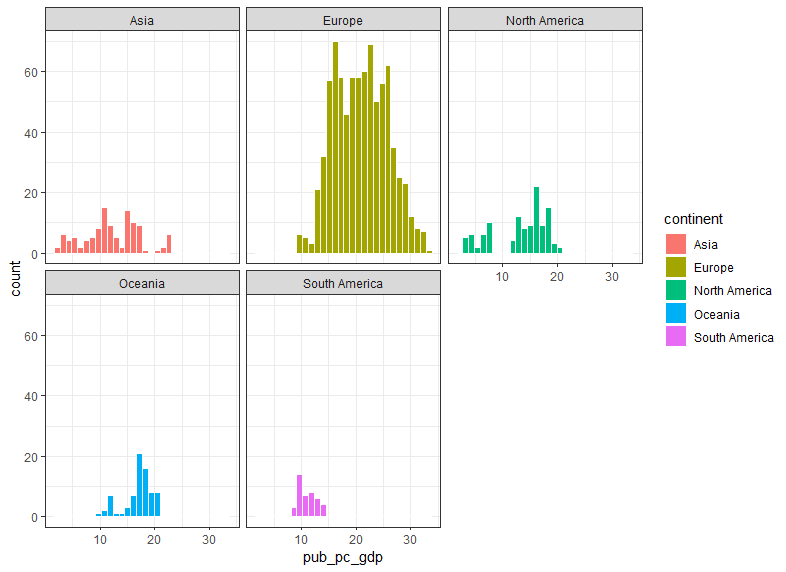I see Europe has higher percentage than other continents about pub_pc_gdp.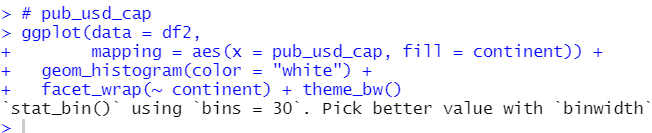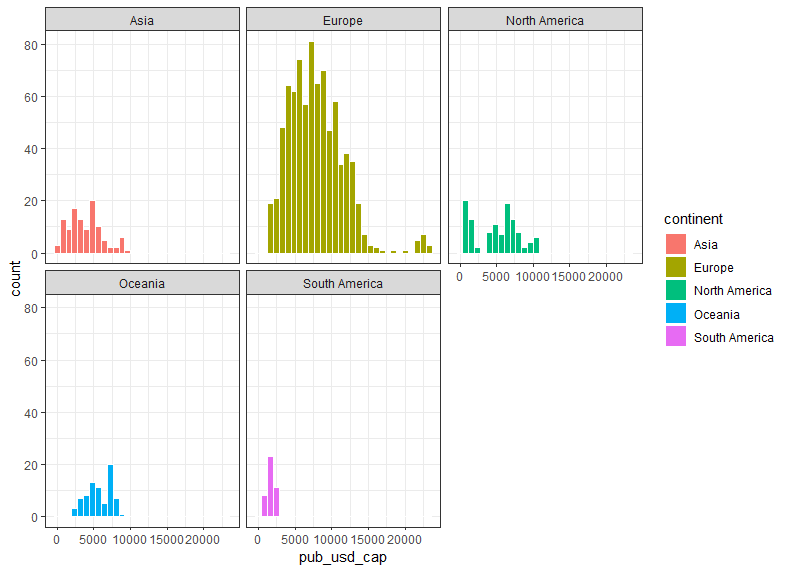Sounth America has the lowest pub_usd_cap distribution.

The 4th 5NG is Boxplot.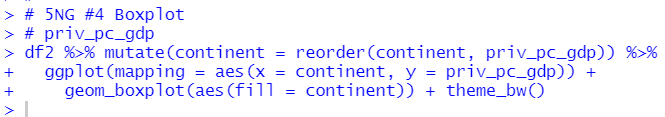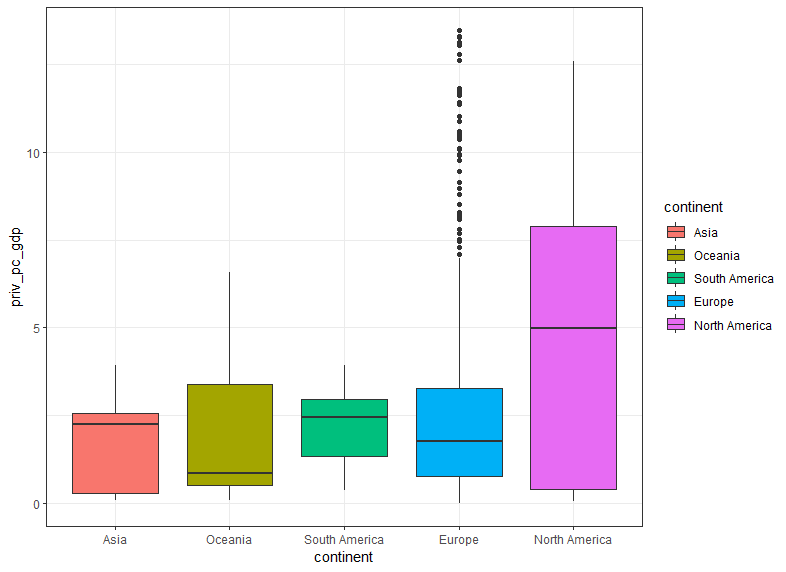geom_boxplot() makes a boxplot. I use mutate() and reorder() to reorder continent by average value. So, I can easily see Notrh America has the highest average priv_pc_gdp.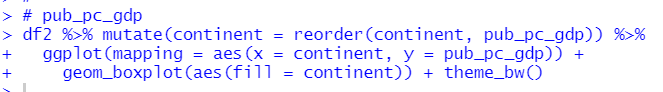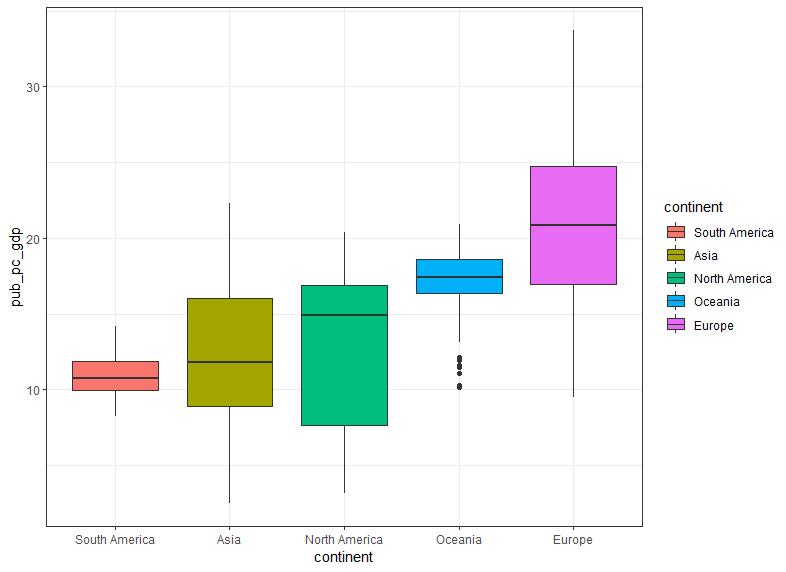I see Europe has the higest average pub_pc_gdp and the highest medain too.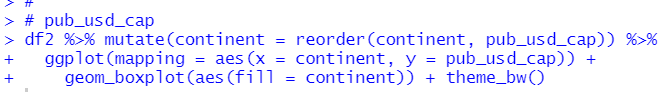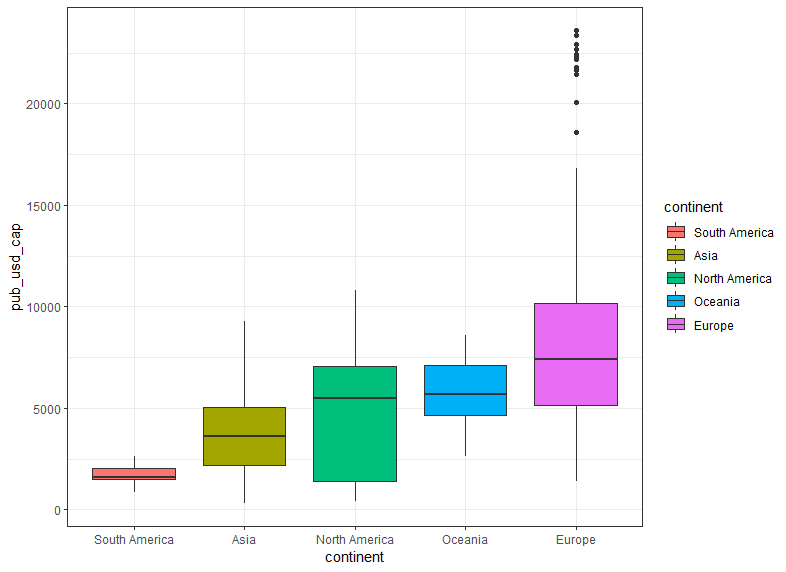Europe has the highest average and median value for pub_usd_cap.

The last 5NG is Barplot.
To make a barplot, we can use geom_bar() and geom_col() function.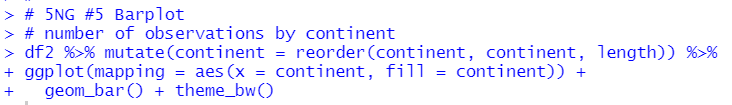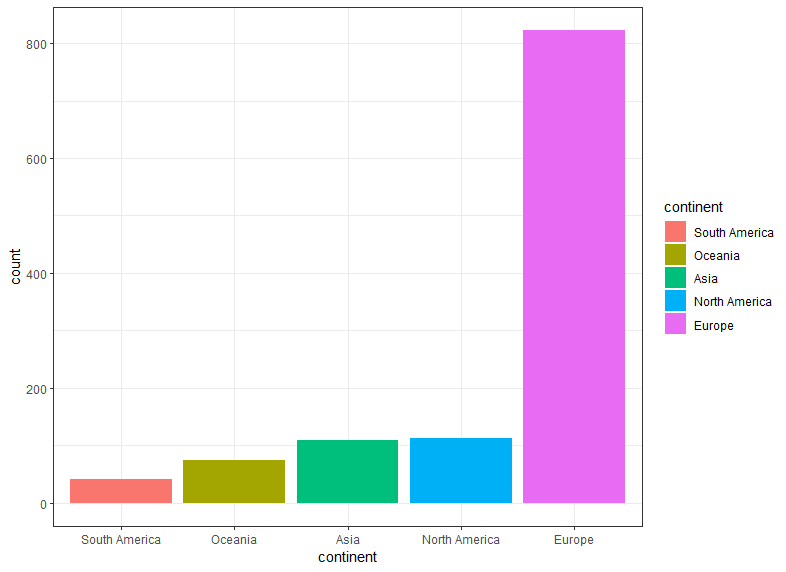geom_bar() automatically count number of observations and make barplot.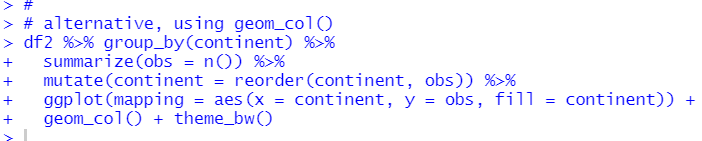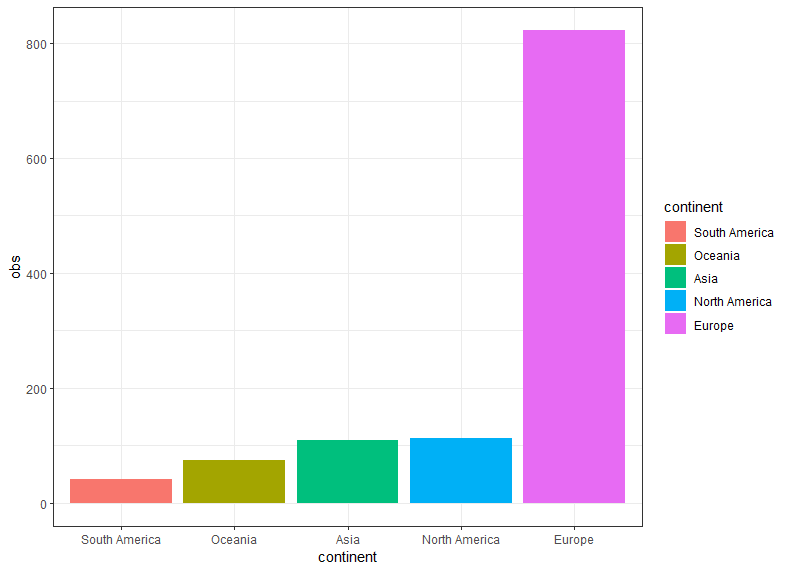Before using geom_col() function, we need to calculate summary statistics data such as number of observations.

That's it. Thank you!

Next post is